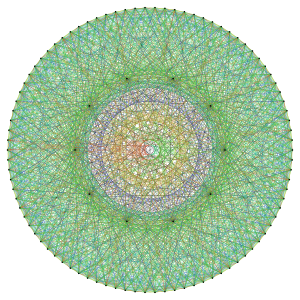## Posts tagged ‘graph isomorphism’

### Graph Isomorphism: Deceptively Hard

This is the first in a series of loosely related posts that will lead up to an announcement of new research results.

Asymptotic complexity is often very useful in succintly expressing how hard a problem is. Occasionally, though, it can obfuscate the picture. Graph isomorphism is a perfect example.

Many people mistakenly believe that graph isomorphism (GI) is hard — either NP-hard, or hard enough to be insoluble in practice for large problem sizes. Certainly the complexity of the best known algorithm — exp(O(sqrt(n log n))), due to Babai and Luks — would appear to support this belief. However, the truth is that GI belongs to its own complexity class, not known to be NP-hard nor known to be soluble in polynomial time. More importantly, on real inputs, graph isomorphism is ridiculously easy. So easy that real-life solvers can plow through hundreds of thousands of instances per second.

Why is this the case? It turns out that on random graphs, GI is solvable in a very straightforward way. You only need to look at the local structure of each node — due to the randomness, every node has a distinctive neighborhood. Thus, the complexity of the worst case input and the average case input are on opposite ends of a spectrum. To see where “real” graphs fall on this spectrum, note that generative models of real graphs have a regular part and a random part. The regular part is captured in rules such as preferential attachment, but such rules only induce a probability distribution; there is still quite a bit of coin tossing needed to generate each edge. Intuitively, if there is “enough” randomness in the neighborhood of each node, then you only need to look at the local structure to tell different nodes apart. Thus, real-world graphs behave pretty much like random graphs.

It gets better: one of the uses of nauty, the leading graph isomorphism solver, is to find hard instances of graph isomorphism. The way this is done is by generating hundreds of candidate hard instances, solving them, and picking the ones that are the hardest to solve. (These are usually highly specialized graphs which are strongly regular and have fearful symmetry groups.) It would appear, then, that finding hard inputs for GI is GI-hard! I wonder if this property can be formally defined, and if there are other known problems for which this is true. This is a similar notion to Impagliazzo’s Heuristica, a world where NP != P, but for any problem in NP, and for inputs drawn from any samplable distribution (i.e, for any “real-world” input), there exists a polynomial time algorithm to decide it.An example of the kind of input that gives graph isomorphism solvers any trouble.

.

While I find this an interesting theory problem, and would love to hear opinions on it from theorists, my reason for posting this is to point out that with all the current evidence, graph isomorphism can be assumed to be solvable in randomized polynomial time for any input that you would actually encounter in reality.

I’m an associate professor of computer science at Princeton. I research (and teach) information privacy and security, and moonlight in technology policy.

This is a blog about my research on breaking data anonymization, and more broadly about information privacy, law and policy.